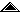Universidad de Costa Rica Escuela de Ciencias de la Computación e Informática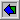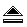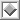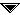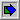# C Iterators

## AbstractC is powerful enough to efficiently access containers through iterators. C es suficientemente poderoso para accesar contenedores eficientemente usando iteradores.

As many others, I have watched C++ grow from cute and slim into a fat computer language. When Stroustrop originally proposed his language, it had no pointers to member, exceptions, templates or namespaces [Str-88], but C++ was nonetheless a huge improvement over C: many useful programs are much shorter if written in C++ instead of C. That is why we deam C++ as a more expressive language. Being a programmer, I always wonder whether all this C++ power is really needed. Besides, given C++'s sheer size, chances are always higher to find a C compiler. This makes it very interesting to implement algorithms in C.

After being exposed to the Standard Template Library [STL], mainly by the detailed articles written by P.J. Plauger, [Pla-96a] & [Pla-96b], I decided to explore the possibility of implementing in C some of the better ideas shown in the STL, using less resources. I thought that this would help in two ways: it could give my readers a better insight on how STL is bolted together, and it could make available STL technology to those who resist using C++ due to its size and complexity.

When faced with scarcity we have to squeeze every ounce of ingenuity to find a solution. That is why I like doing things the hard way, to get a better insight in how to achieve results, and oftentimes to find a more efficient solution.. Recall that Alexander Stepanov, the STL's main architect, had the opportunity to change C++ to acomodate for his special needs [Ste-95]. Maybe a less favorable environment would have lead to a slimmer C++.

## Printing a list```void traverse (list *L, iterator(list)* I) { for (I->bind(I, L); !I->finished(I); I->next(I)) { long *v = (long*) list_retrieve(L, I->here(I)); printf(" %ld", *v); } } /* traverse */ ```
Figure 1: Printing a list

Listing 1 is my implementation of program `c-iter.c`, that uses a parametrized list and a few iterators. The main work is done in routine `traverse(&L,&I)`, also shown in Figure 1, where list "`L`" gets printed in the order determined by iterator "`I`". In case you have not heard, an iterator is just a smart pointer into a container; iterators are usefull because they provide efficient access to the values stored in the container, but relieve the programmer from knowing the innards of the implementation.

Look into the implementation of `traverse(&L,&I)` and you will see that it has only a `for(;;)` cycle where four functions are invoked, each of which has the usual role in these type of cycle:

 1 Setup: `I->bind()` 2 Cycle condition: `I->finished()` 3 Advance: `I->next()` 4 Use value: `I->here() `

 ```for (i=0; i
Figure 2: Printing array `A[]`

Figure 2 is the code used to print the values stored in array `A[]`. Compare this code with the implementation of `traverse(&L,&I)` and you will notice that these two `for(;;)` cycles are pretty similar. This fact is made explicit in the first columns in Table 1.

 Setup `i = 0;` `I->bind(I, L);` `itr_bind(I, L);` Cycle condition `ifinished(I);` `!itr(I,finished);` Advance `i++` `I->next(I)` `itr(I,next)` Use value `A[i]` `I->here(I)` `itr(I,here)`
Table 1: Iterators and arrays

When run, `c-iter` will store some values in list "`L`", and then print them in different orders. Each iterator provides access to "`L`" in a different way: "`Iforw`" traverses "`L`" from its first value to the last, whereas "`Iback`" goes from the last to the first. The last iterator, "`Iorder`", yields all the values in order, from smaller to bigger. What makes `traverse()` interesting is that the same function will yield values in different orders: this is code reutilization, the polimorphic way.

The trick used to change dramatically `traverse()`'s behaviour is to use function pointers. Hence, the code `I->next(I)` actually invokes the function pointed to by field "`next`" stored within "`*I`"; when a different iterator is used, it will contain a different function pointer, and thus `traverse()`'s behaviour would be changed: no black magic, just pointer juggling.

## A list classI had to implement a list class in C to use with these iterators. I chose the implementation that would require less code, even though my favority has always been the circular singled linked list, because it lets you append and prepend in constant time. Listing 2 is the header file `list.h`, and Listing 3 is its implementation `list.c`. As usual, this linked list is implemented using nodes where values get stored. I use pointer type "`lpos`" to shield the client programmer of `list.h` from the implementation. This means that the list operations, for example `list_count()` or `list_append()`, take pointer arguments of type "`lpos`", but such a pointer cannot be used to access a stored value by itself: it must be typecasted into a node pointer, of type "`list_node`".

All this might seem strange, but I tried to make `list` into a trully polimorphic and parametric type, meaning that lists that contain different element types will share the same implementation. This is a contrast to using a C++ `<list>` template, because template instanciation usually yields different versions of the same algorithm for each element type. When initialized with `init_list()`, any `list` variable must be passed the element size, which will be later used to create a node big enough to hold the linked list pointer "`next`", and the element value. This implementation is not complete, because it will not handle element types that require special construction or destruction, but in good enough form many applications. The price paid to achieve polymorphism is lack of type checking, because the list operations that store values take typeless arguments (`void*`).

A function defined in `list.h` that deserves special discussion is `list_retrieve(&L,p)`, because it transforms a list position "`lpos`", into a pointer to the stored value in a node. It returns a typeless pointer (`void*`) because lists contain elements of unknown type, and it is up to the programmer to typecast this pointer into the proper pointer type. This explains why in the implementation of `traverse()` in Figure 1, the value returned by `I->here()` must be typecasted explicitely into a `(*long)` before using it.

I included just enough operations in `list.h` to have the code compile and run. Some of the opertions in `list.h` are implemented as macros, to achieve the efficiency of C++ inline functions. You can download all this code, including another list implementation that uses arrays instead of node pointers.

## IteratorsAs C lacks Object Oriented Programming [OOP] facilities, it is oftentimes difficult to express some algorithms. I had to use the macro processor to overcome this restriction, following a bit the approach sugested in [BSG-92], the result being header file `iterator.h`, shown in Listing 4. In there, macro `itr()` is defined so that the invocation:
`!itr(I,finished);`
translates into:
`!I.vmt.finished(&I.vmt);`
which invokes the "`finished()`" iterator function pointed to by iterator "`I`". Every iterator contains a field, called "`vmt`", where all the pointers to iteration functions are stored. In OOP parlance, VMT stands for "Virtual Method Table", which is a vector of pointer to functions. The macro `define_itr_vmt()` is used to define all the pointer fields that point into the iterator operations.

Listing 5 is the header file `forwl.h`, that contains the definition for the "`list_forward`" iterator type; its implementation is shown as Listing 6. Macro invocation `itr_vmt(list)` is used to define the iterator's "`vmt`" field. Other fields are a pointer to the list, and a "`lpos`" marking the iterator's current position in the list. It is necessary to keep a pointer to the list because, for brevity and easy of use, the iterator operations `finished()`, `here()`, etc., do not take a list argument. For this definition of `list_forward` a "`typedef struct`" is used because C lacks classes, which makes mandatory to use `typedef` to avoid carrying around the keyword `struct` when declaring aggregate fields like "`vmt`".

 ```typedef struct itr_list_vmt_ { void (*bind) (struct itr_list_vmt_ *, list*); int (*finished) (struct itr_list_vmt_ *); lpos (*here) (struct itr_list_vmt_ *); void (*next) (struct itr_list_vmt_ *); void (*done) (struct itr_list_vmt_ *); } itr_list_vmt; typedef struct list_forward_ { list * L; lpos p; /* here() */ itr_list_vmt vmt; } list_forward; ```
Figure 3: Fields for iterator `list_forward`

The result of the macro invocations used to define the fields in a forward iterator is shown in Figure 3. Field "`vmt`" contains all the function pointers to run the iterator, while the other fields are used to store its current state.

 ```void print_list(list* L) { list_forward Iforw; init_forward(&Iforw); printf("\n\n ==== forward ======\n"); for ( itr_bind(Iforw, *L); /* i = 0 */ !itr(Iforw,finished); /* i < n */ itr(Iforw,next) /* i++ */ ) { long *v = (long*) list_retrieve(&L, itr(Iforw, here)); printf(" %ld", *v); } done_forward( &Iforw.vmt ); } ```
Figure 4: Direct use of an iterator

If you are of the observing type, by now you would have noticed that, in program `c-iter.c` (see Figure 4), sometimes the iterator itself is used as an argument, as in
`init_forward( &Iforw );`
whereas in other cases the iterator's VMT is used instead:
`done_forward( &Iforw.vmt );`

Why this disparity? The answer lies in C's lack of support for OOP. In any language that supports inheritance, every iterator would be derived from a general "`Iterator`" class. To fake the same in C, in every iterator instance we need to include a field, precisely "`vmt`", where the common inherited fields get stored. Hence, we achieve the effect of inheritance by passing around as argument the common "`vmt`" field. This also explains the need to invoke macro `vmt_self()` in the implementation of each iterator operation. Look, for example, into the implementation of `finished_forward()` in Listing 6, where the pointer to the iterator VMT "`&I.vmt`" is transformed into a pointer to the iterator itself "`&I`" at the very beginning:
`list_forward *I = vmt_self(list_forward, I_vmt);`
From there on, "`I`" points to a full "`list_forward`", that contains both "`I->p`" and "`I->L`" besides the "`I->vmt`" field.

## Usage stylesTo use an iterator in a program, the easier way is to invoke its operations through the `itr()` macro, defined in `iterator.h`. However, to pass it around as a polymorphic argument, it is necessary to use the "`vmt`" field.

Figure 4 is the usual usage of an iterator. After initializing it, macro `itr()` is used to invoke each of the iteration operations. As the list is typeless, the programmer must take special care to convert the position pointers returned by the `here()` into the proper value pointer.

The other usage style was used to implement `traverse()` as in Figure 1. The iterator's "`vmt`" field is passed as the argument, and inside the function a different syntax is required to access the iterator operations, as is shown in the last two columns of Table 1. The syntax requires naming the iterator twice: one to access the pointer to function field, and the other to pass the iterartor itself; in any OOP language, the later is the C++ "`this`" pointer. To my taste, the code does not look that bad, but you could always define (yet) another macro in `iterator.h` to avoid this duplicity.

In OOP languajes each object instance does not contain a full copy of the VMT, as it is the case in my implementation, but a pointer to a shared VMT. I decided to ease up on this, as not that many iterators are used in a program, which makes the increased storage requirements of my implementation negligible. Besides, in this way I am saving the extra pointer indirection required to jump from the "`vmt`" field pointer to the actual VMT table.

I must add that my iterators differ a bit from STL iterators in that I do not explicitly provide output iterators, this is, iterators used to store more values in the container. I believe that an iterator should never change the value stored in the container, but the architects of the STL had a different opinion. Besides, C does not have enough expresive power to use output iterators in a meaningful way, as opossed to C++, where they can be used to load the container seemlessly from a string, or from another container.

## Implementing other iteratorsListing 7 and Listing 8 are the definition and implementation for the "`order`" iterator. There are some implicit rules to follow when naming each of the iterator operations, as otherwise the macros in `iterator.h` would not function properly. As C does not have name overloading, we need to prepend the name of the container, "`list`" in this case, to the name of the iterator, "`order`", to obtain the full iterator type name: "`list_order`". Also, the name of each operation includes, at the end, the name of the iterator. For example, the `bind()` operation for "`order`" is called "`bind_order()`".

In the definition of each operation I used the macro `iterator()`, defined in `iterator.h`, that yields the type of its "`vmt`" field. This is the field macro `vmt_self()` works on, by getting a pointer to the whole iterator from a pointer to its VMT.

In iterator `order`'s implementation I use a vector of list positions, which I bubble sort. When `bind_order(&L, &I.vmt)` is invoked, it will allocate an array where one "`lpos`" would be stored for each element in the list; this is the array that gets sorted. The purpose of "`bind()`" is to associate the iterator with its container. Note that operation `next_order()` advances in this vector but, when it gets past the end, the dynamic memory in used by the iterator is immediately released. Hence, it is improper to invoke `here_order()` when `finished_order()` no longer returnes FALSE, because the vector of positions would no longer be available.

Note also that operation `finished()` can be called as many times as needed, as it never changes the value of the iterator: that task is reserved for `bind()`, that sets the iterator to its first position, and `next()`, that moves on forward.

Even though these iterators are quite efficient, they do not really access `list`'s private data fields. For example, if the list where implemented as an array, and each position `lpos` where an array index, then the same implementation for each each of the iterators would work seemlessly with this other `list` type. Due to space limitations, this other list implementation did not get printed, but nonetheless keep in mind that you will not always need to break the data abstraction to achieve efficiency: do it only when required.

An iterator always does a lot of pointer juggling. Examine carefully the bubble sort in `bind_order()`, that transforms positions into value pointers, to later invoke a function to tell whether the corresponding values are in order. A special comparison function, `I->fcmp()`, must be provided when the iterator is initialized, through `init_order()`. Its definition is similar to the comparison function that the standard `qsort()` receives as its last argument. Note that list positions are first transformed into pointed values by invoking `list_retrieve()`, and then those are the pointers handed to the comparison function `I->fcmp()`.

After implementing iterator "`order`", I set up to implement "`backl`", to traverse the list backward. I used a vector as in "`order`", but this time instead of ordering the position pointers, I just stored them backwards. Because of this, I just copied most of the operation implementations from "`order.c`" into "`backl.c`", but I had to twickle `bind_backward()`. I also had to change some identifiers from "order" to "backward".

You can download all the code in this article from the Internet, including that not printed. If you are typing the code, and you did not get yet the backward iterator, you can nevertheless compile "`c-iter.c`" by commenting out the following line:
`#include "backl.h"`

## ConclusionA little macro tweaking with some pointer jugging yields iterators good enough for most applications. It is always better to implement them in an OOP language, like Embedded C++ [Pla-97] or C++, but with a little care a programmer can build a container library in C that is efficient and provides some of the better features found in more complicated libraries, like the C++ STL. You can download all the code in this article from:
``` http://www.di-mare.com/adolfo/p/src/c-iter.zip```

## AcknowledgmentsBoth Ronald Argüello and Carlos Loría took the time to critizice and earlier version of this paper. Later, Bjarne Stroustrup told me that only when forced should one decide to use C instead of C++, and that STL iterators are more efficient than the ones I present here. I agree with him.

This research was made in project 326-98-391 "Polimorfismo uniforme más eficiente", funded by Vicerrectoría de Investigación in the Universidad de Costa Rica. The Escuela de Ciencias de la Computación e Informática has also provided funding for this work.

## Bibliography[BSG-92] Bingham, Bill & Schlintz, Tom & Goslen, Greg: OOP Without C++, C/C++ User's Journal, Vol.10, No.3, pp [31, 32, 34, 36, 39, 40], March 1992. [Pla-96a] Plauger, P. J.: The Header ``, Part 1, C/C++ User's Journal, Vol.14, No.4, pp [8, 10, 12, 14, 16], April 1996. [Pla-96b] Plauger, P. J.: The Header ``, Part 2, C/C++ User's Journal, Vol.14, No.5, pp [8, 10, 12, 14-16], May 1996. [Pla-97] Plauger, P. J.: Embedded C++, C/C++ Users Journal, Vol.15 No.2, pp [35-39], February 1997. [Ste-95] Stevens, Al: Alexander Stepanov and STL, Dr. Dobbs's Journal, No.228, pp [118-123], March 1995.       `http://www.sgi.com/Technology/STL/drdobbs-interview.html` [Str-88] Stroustrup, Bjarne: What is Object-Oriented Programming, IEEE Software, pp [10-20], May 1988.       `http://www.research.att.com/~bs/whatis.ps`

## Listing 1: `c-iter.c````/* c-iter.c v0.1 (C) 1999 adolfo@di-mare.com */ /* compile and link together: c-iter+list ==> main && container forwl+backl+order ==> iterators */ #include "list.h" #include "iterator.h" #include "forwl.h" #include "backl.h" #include "order.h" #include #include #include void traverse (list *L, iterator(list)* I); void print_list(list* L); void traverse (list *L, iterator(list)* I) { for (I->bind(I, L); !I->finished(I); I->next(I)) { long *v = (long*) list_retrieve(L, I->here(I)); printf(" %ld", *v); } } /* traverse */ void main () { unsigned long memInit = coreleft(); list L; list_init(L, long); { int i; srand(0); printf("\n\n ==== loading ======\n\n"); for (i=1; i<=23; i++) { long val; val = i; val = rand(); list_append(&L, &val); printf(" %ld", val); } } { lpos p; printf("\n\n ==== nextnext======\n\n"); for (p = list_first(&L); NULL != p; p = list_next(&L,p)) { long *v = (long*) list_retrieve(L, p); printf(" %ld", *v); } } { list_forward Iforw; init_forward(&Iforw); printf("\n\n ==== forward ======\n"); traverse(&L, &Iforw.vmt); done_forward( &Iforw.vmt ); } print_list(&L); { int longcmp(const void *a, const void *b); list_order Iorder; init_order(&Iorder, longcmp); printf("\n\n ==== order ======\n"); traverse(&L, &Iorder.vmt); done_order (&Iorder.vmt ); } { #ifdef BACKL_H list_backward Iback; init_backward(&Iback); printf("\n\n ==== backward ======\n"); traverse(&L, &Iback.vmt); done_backward(&Iback.vmt ); #endif } list_done(&L); if (memInit != coreleft()) { printf("\n ==== memory allocation error ====\n"); }; } void print_list(list* L) { list_forward Iforw; init_forward(&Iforw); printf("\n\n ==== forward ======\n"); bind_forward(&Iforw.vmt, L); (Iforw.vmt).bind(&(Iforw.vmt), L); for ( itr_bind(Iforw, *L); /* i = 0 */ !itr(Iforw,finished); /* i < n */ itr(Iforw,next) /* i++ */ ) { long *v = (long*) list_retrieve(L, itr(Iforw, here)); printf(" %ld", *v); } done_forward( &Iforw.vmt ); } int longcmp(const void *a, const void *b) { if ( *((long*)a) < *((long*)b) ) { return -1; } else if (*((long*)a) > *((long*)b)) { return 1; } else { return 1; } } /* EOF: c-iter.c */ ```

## Listing 2: `list.h````/* list.h v0.1 (C) 1999 adolfo@di-mare.com */ #ifndef LIST_H #define LIST_H #ifdef __cplusplus extern "C" { #endif #include typedef struct { char nada; } *lpos; typedef struct { lpos _first; /* private */ size_t _node_size; /* private */ } list, *plist; #define list_init(L, VTYPE) \ list_init_(&L, sizeof(VTYPE)) void list_done(list *); size_t list_count(list *L); void list_insert(list *, lpos, void*); #define list_append(L, val) \ list_insert(L, list_last(L), val) #define list_first(L) ((L)->_first) lpos list_last(list *L); #define list_next(L,p) ((lpos) (((list_node*)p)->next)) /* convert to (void) pointer to stored value */ #define list_retrieve(L,p) \ ((void*) & ((list_node*) p)->val) /* Non-macro versions of inlined code */ void list_init_ (list *, size_t); void list_append_ (list *, void*); lpos list_first_ (list *); lpos list_next_ (list *, lpos); void * list_retrieve_ (list *, lpos p); typedef struct list_node_ { struct list_node_ * next; /* private */ char val; /* private */ } list_node; #ifdef __cplusplus } #endif #endif /* LIST_H */ /* EOF: list.h */ ```

## Listing 3: `list.c````/* list.c v0.1 (C) 1999 adolfo@di-mare.com */ #include "list.h" #include #include typedef list_node node; #define lpos_node(p) ((node*)p) void list_init_(list *L, size_t sz) { L->_node_size = sz + offsetof(list_node, val); L->_first = NULL; } void list_done(list *L) { /* destructor */ while (NULL != lpos_node(L->_first)) { node *next = lpos_node(L->_first)->next; /* a better implementation would invoke the destructor node->~val() */ free(L->_first); lpos_node(L->_first) = next; } } size_t list_count(list *L) { list_node *p = (list_node*) (L->_first); size_t count = 0; while (NULL != p) { p = p->next; count++; } return count; } void list_insert(list *L, lpos p, void *val) { /* Puts "val" after position "p" in list "L" - Not trully generic: copies "*val" with memcpy() instead of val.Operator=() */ /* allocate just enough storage for "*val" */ node * new_node; new_node = (node *) malloc(L->_node_size); /* should use val.Operator=() */ memcpy( &new_node->val, val, L->_node_size-offsetof(list_node, val)); if (NULL == p) { new_node->next = (node*)(L->_first); L->_first = (lpos) new_node; } else { new_node->next = ((node*)p) -> next; ((node*)p)->next = new_node; } } lpos list_last(list *L) { node *last = (node*) (L->_first); if (NULL != last) { while (NULL != last->next) { last = last->next; } } return (lpos) last; } #ifdef COMPILE_NON_INLINE_METHODS /* Non-macro versions of inlined code */ void list_append_(list *L, void *val) { /* inserts at the end of the list */ list_insert(L, list_last(L), val); } lpos list_first_(list *L) { return L->_first; } void* list_retrieve_(lpos p) { #pragma argsused return list_retrieve(L,p); } lpos list_next_(list *L, lpos p) { #pragma argsused return ((lpos) ( ((list_node*)p)->next)); } #endif /* EOF: list.c */ ```

## Listing 4: `iterator.h````/* iterator.h v0.1 (C) 1999 adolfo@di-mare.com */ #ifndef ITERATOR_H #define ITERATOR_H #include /* offsetof() */ #ifdef __cplusplus extern "C" { #endif #define define_itr_vmt(CONTAINER, CPos) \ typedef struct itr_ ## CONTAINER ## _vmt_ { \ \ void (*bind) (struct itr_ ## CONTAINER ## _vmt_ *, CONTAINER*);\ int (*finished) (struct itr_ ## CONTAINER ## _vmt_ *); \ CPos (*here) (struct itr_ ## CONTAINER ## _vmt_ *); \ void (*next) (struct itr_ ## CONTAINER ## _vmt_ *); \ void (*done) (struct itr_ ## CONTAINER ## _vmt_ *); \ } itr_ ## CONTAINER ## _vmt #define itr_vmt(CONTAINER) \ itr_ ## CONTAINER ## _vmt vmt #define iterator(CONTAINER) \ itr_ ## CONTAINER ## _vmt #define itr_bind(itr, container) \ (itr).vmt.bind(&((itr).vmt), &(container)) #define itr(I, method) ((I).vmt.method(& ((I).vmt) )) typedef struct { char v[ (sizeof(char)==1 ? 1 : 0) ]; } check_that_size_of_char_is_one_; #define vmt_self(ITR_TYPE, ITR) \ ((ITR_TYPE *) (((char *)ITR) - offsetof(ITR_TYPE, vmt))) /* +----+ */ /* This requires sizeof(char) == 1 */ typedef struct iterator_vmt_ { void (*bind) (struct iterator_vmt_ *, void *); int (*finished) (struct iterator_vmt_ *); void * (*here) (struct iterator_vmt_ *); void (*next) (struct iterator_vmt_ *); void (*done) (struct iterator_vmt_ *); } iterator_vmt; #ifdef __cplusplus } #endif #endif /* ITERATOR_H */ /* EOF: iterator.h */ ```

## Listing 5: `forwl.h````/* forwl.h v0.1 (C) 1999 adolfo@di-mare.com */ /* Traverses a list forward, from its first to its last element */ #ifndef FORWL_H #define FORWL_H #ifdef __cplusplus extern "C" { #endif #include "list.h" #include "iterator.h" #ifndef iterator_list_ define_itr_vmt(list, lpos); #define iterator_list_ #endif typedef struct list_forward_ { char mark; list * L; lpos p; /* here() */ itr_vmt(list); } list_forward; void init_forward(list_forward *); void bind_forward( iterator(list) *I , list *L); int finished_forward( iterator(list) *I ); lpos here_forward( iterator(list) *I ); void next_forward( iterator(list) *I ); void done_forward( iterator(list) *I ); #ifdef __cplusplus } #endif #endif /* FORWL_H */ /* EOF: forwl.h */ ```

## Listing 6: `forwl.c````/* forwl.c v0.1 (C) 1999 adolfo@di-mare.com */ #include "forwl.h" #include void init_forward(list_forward *I) { memcpy(I->mark, "Init", 12); I->vmt.bind = bind_forward; I->vmt.finished = finished_forward; I->vmt.here = here_forward; I->vmt.next = next_forward; I->vmt.done = done_forward; I->L = NULL; I->p = NULL; } void bind_forward( iterator(list) *I_vmt , list *L) { list_forward *I = vmt_self(list_forward, I_vmt); memcpy(I->mark, "bind", 12); I->p = list_first(&(*L)); I->L = L; } int finished_forward( iterator(list) *I_vmt ) { list_forward *I = vmt_self(list_forward, I_vmt); memcpy(I->mark, "finished", 12); return (NULL == I->p); } lpos here_forward( iterator(list) *I_vmt ) { list_forward *I = vmt_self(list_forward, I_vmt); memcpy(I->mark, "here", 12); return I->p; } void next_forward( iterator(list) *I_vmt ) { list_forward *I = vmt_self(list_forward, I_vmt); memcpy(I->mark, "next", 12); I->p = list_next(I->L, I->p); } void done_forward( iterator(list) *I_vmt ) { #pragma argsused /* do nothing destructor */ } /* EOF: forwl.c */ ```

## Listing 7: `order.h````/* order.h v0.1 (C) 1999 adolfo@di-mare.com */ /* Traverse a list in order, from the smaller to the bigger elements */ #ifndef ORDER_H #define ORDER_H #ifdef __cplusplus extern "C" { #endif #include "list.h" #include "iterator.h" #ifndef iterator_list_ define_itr_vmt(list, lpos); #define iterator_list_ #endif typedef struct list_order_ { char mark; itr_vmt(list); list * L; lpos * v; size_t index, count; int (*fcmp)(const void *, const void *); } list_order; void init_order(list_order *, int (*fcmp)(const void *, const void *)); void bind_order( iterator(list) *I , list *L); int finished_order( iterator(list) *I ); lpos here_order( iterator(list) *I ); void next_order( iterator(list) *I ); void done_order( iterator(list) *I ); #ifdef __cplusplus } #endif #endif /* ORDER_H */ /* EOF: order.h */ ```

## Listing 8: `order.c````/* order.c v0.1 (C) 1999 adolfo@di-mare.com */ #include "order.h" #include #include void init_order( list_order *I, int (*fcmp)(const void *, const void *) ) { memcpy(I->mark, "Init", 12); I->vmt.bind = bind_order; I->vmt.finished = finished_order; I->vmt.here = here_order; I->vmt.next = next_order; I->vmt.done = done_order; I->L = NULL; I->v = NULL; I->fcmp = fcmp; } void bind_order( iterator(list) *I_vmt , list *L) { list_order *I = vmt_self(list_order, I_vmt); memcpy(I->mark, "bind", 12); if (NULL != I->v) { free(I->v); } I->index = 0; I->count = list_count(L); if (0 == I->count) { I->v = NULL; return; } I->L = L; I->v = (lpos *) malloc(I->count * sizeof(lpos)); { int i = I->count; lpos p = list_first(L); while (i>0) { i--; I->v[i] = p; p = list_next(L, p); } } { int changed; size_t j, k, N = I->count; k = 0; do { /* yep: bubble sort! */ changed = 0; for (j = 0; (j < N-k); j++) { void *vj = list_retrieve(L, I->v[j]); void *vjplus = list_retrieve(L, I->v[j+1]); if (I->fcmp(vj, vjplus) > 0) { lpos tmp = I->v[j]; I->v[j] = I->v[j+1]; I->v[j+1] = tmp; changed = 1; } } k++; } while (0 != changed); } } int finished_order( iterator(list) *I_vmt ) { list_order *I = vmt_self(list_order, I_vmt); memcpy(I->mark, "finished", 12); return (NULL == I->v); } lpos here_order( iterator(list) *I_vmt ) { list_order *I = vmt_self(list_order, I_vmt); memcpy(I->mark, "here", 12); return I->v[I->index]; } void next_order( iterator(list) *I_vmt ) { list_order *I = vmt_self(list_order, I_vmt); memcpy(I->mark, "next", 12); I->index++; if (I->index >= I->count) { free(I->v); I->v = NULL; } } void done_order( iterator(list) *I_vmt ) { list_order *I = vmt_self(list_order, I_vmt); memcpy(I->mark, "done", 12); if (NULL != I->v) { free(I->v); } } /* EOF: order.c */ ```

## Index[-] Abstract [-] Listing 1: `c-iter.c` [-] Printing a list [-] Listing 2: `list.h` [-] A list class [-] Listing 3: `list.c` [-] Iterators [-] Listing 4: `iterator.h` [-] Usage styles [-] Listing 5: `forwl.h` [-] Implementing other iterators [-] Listing 6: `forwl.c` [-] Conclusion [-] Listing 7: `order.h` [-] Acknowledgments [-] Listing 8: `order.c`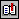BibliographyIndex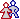About the author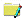About this documentTopIndexBottom

## About this documentReference: Di Mare, Adolfo: C Iterators, Revista Acta Académica, Universidad Autónoma de Centro América, Número 26, pp [14-30], ISSN 1017-7507, Mayo 2000. Internet: ``` http://www.uaca.ac.cr/actas/2000may/c-iter.htm ``` ``` http://www.di-mare.com/adolfo/p/c-iter.htm``` ``` http://www.di-mare.com/adolfo/p/src/c-iter.zip ``` Author: Adolfo Di Mare ``` ``` Contact: Apdo 4249-1000, San José Costa Rica Tel: (506) 207-4020       Fax: (506) 438-0139 Revision: ECCI-UCR, May 2000 Visitors: